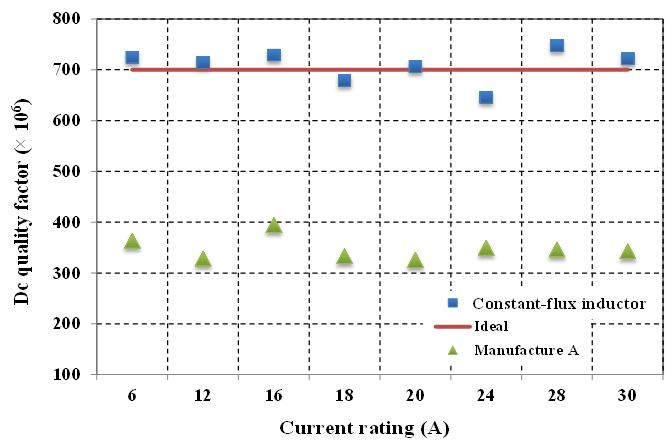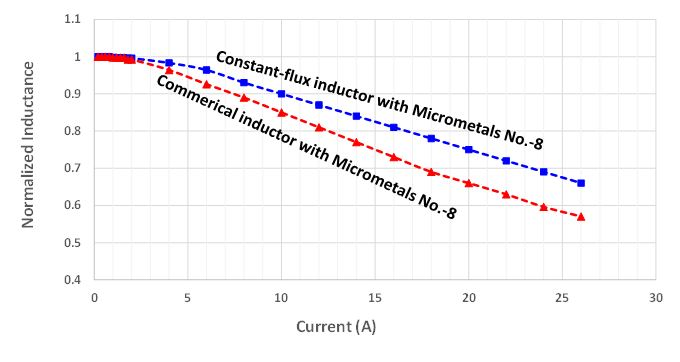LIBRARY

# Design and Evaluation of the Constant-Flux Inductor with Enclosed Winding

Year: 2015Fig. 1. Comparison of dc quality factor between a series of commercial inductors with a package size of 10 10 4 mm3 and constant-flux inductors with same inductance and package size for different current ratings
The recently introduced "constant-flux" concept is leveraged to distribute magnetic flux in order to improve energy density, lowering the profile of an inductor. The optimal flux distribution with normalized parameters is identified mathematically, and verified by simulation. It is then applied to reduce the DC resistance of a commercial inductor by a factor of two, keeping the outer dimensions and inductance the same. Thermal-limited current rating is improved by 50%, where-as saturation-limited current rating is improved by 20% thanks to the suppression of flux crowd-ing. The simulation result is verified by measurement results of a prototype under high current bias.

For this paper, a behavioral model of a constant-flux inductor was constructed. With given specifications, a winding configuration with uniform flux distribution can be achieved by following the design equations and procedures. In order to generalize the design procedure and to analyze the impacts of design parameters systematically, the constructed model with normalized parameters is discussed herein. As shown in Fig. 1, by using normalized parameters for an optimal design, an improvement factor of two on the Qdc is obtained for the whole series of inductors thanks to the constant-flux distribution.

In this paper, the optimal design of a constant-flux inductor that maximizes the inductor's performance is discussed. The design procedure of the constant-flux inductor is normalized so that the results are more general. In addition, the normalization decouples geometrical dimensions and magnetic material properties in design and evaluation. The heat rating current is improved by 50% because the DC quality factor of the constant-flux inductor is two times larger in comparison to a commercial product with the same volume and inductance. The saturation rating current is also improved by 20% as a result of the uniform flux distribution inside the core. The simulation result of the saturation current is verified by the measurement results on the fabricated inductor prototype under different DC currents.Fig. 2. Comparisons of normalized inductance under different current excitation with Micrometals No. -8 material for commercial inductor and constant-flux inductor